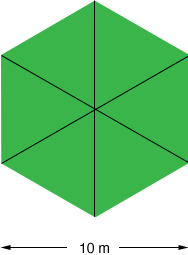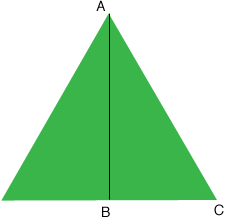Quandaries and Queries I have a perfect Hexagon in which i know the measurement across is 10m. Not from point to point, but from the middle of one side directly across to the middle of the other. From that measurement, how can I determine the length of 1 side. In case your wondering about the application, I am a Glazier and run into people with a broken patio table glass that is a hexagon shape. The reason i can't get a corner to corner measurement from the frame is because the corners are usually rounded. Any response on this would be appreciated. Hi Timothy, If you draw the three diagonals in a regular hexagon the hexagon is divided into six equilateral triangles.Since your hexagon is 10 meters across, each of these triangles has a height of 5 meters. I drew one of these triangles below.The length of the line AB is 5 meters and, since the tiiangle is equilateral, the angle BCA measures 60o. Thus |AB|/|AC| = sin(60o) = sqrt(3)/2 Thus |AC| = 2|AB|/sqrt(3) = 10/sqrt(3) = 5.7735 meters Thus the length of each side of the hexagon is 5m 77cm and 35mm. I think there must be a typo in your statement of the problem. I doubt that you have a patio table that measures 10m across. The solution above however tells you how to find the length of a side of a regular hexagon. Take the measurement across (from the middle of one side directly across to the middle of the other) and divide by the square root of 3. Penny Go to Math Central